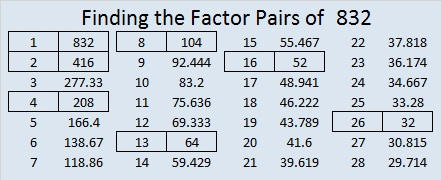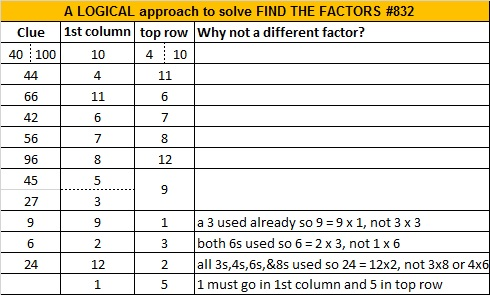# 832 and Level 4Print the puzzles or type the solution on this excel file: 12 factors 829-834

832 has many factors, but it can be written as the sum of consecutive numbers only one way:

• 58 + 59 + 60 + 61 + 62 + 63 + 64 + 65 + 66 + 67 + 68 + 69 + 70 = 832; that’s thirteen consecutive numbers.

832 can be written as the difference of two squares five different ways because it has five factor pairs in which both numbers are even:

• 416 × 2 = 832 means 209² – 207² = 832
• 208 × 4 = 832 means 106² – 102² = 832
• 104 × 8 = 832 means 56² – 48² = 832
• 52 × 16 = 832 means 34² – 18² = 832
• 32 × 26 = 832 means 29² – 3² = 832

832 is also the sum of two squares:

• 24² + 16² = 832

832 is the hypotenuse of a Pythagorean triple:

• 320-768-832 calculated from 24² – 16², 2(24)(16), 24² + 16²
• 320-768-832 is also 64 times (5-12-13)

832 is repdigit QQ in BASE 31 (Q is 26 base 10). That’s because 26(31) + 26(1) = 832, which is the same as saying 26 × 32 = 832.

• 832 is a composite number.
• Prime factorization: 832 = 2 × 2 × 2 × 2 × 2 × 2 × 13, which can be written 832 = 2⁶ × 13
• The exponents in the prime factorization are 6, and 1. Adding one to each and multiplying we get (6 + 1)(1 + 1) = 7 × 2 = 14. Therefore 832 has exactly 14 factors.
• Factors of 832: 1, 2, 4, 8, 13, 16, 26, 32, 52, 64, 104, 208, 416, 832
• Factor pairs: 832 = 1 × 832, 2 × 416, 4 × 208, 8 × 104, 13 × 64, 16 × 52, or 26 × 32
• Taking the factor pair with the largest square number factor, we get √832 = (√64)(√13) = 8√13 ≈ 28.8444102This site uses Akismet to reduce spam. Learn how your comment data is processed.